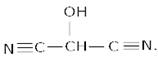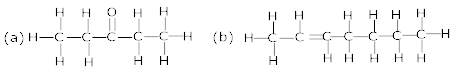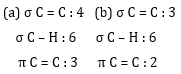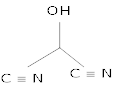By | September 23, 2020

CBSE TEST PAPER 01

CLASS XI CHEMISTRY (Organic Chemistry Some Basic Principle and Techniques)

General Instruction:

• All questions are compulsory.
• Marks are given alongwith their questions.

1. Write the expanded form of the following condensed formulas into their complete structural formulas.
(a) CH3CH2COCH2CH3.
(b) CH3CH=CH(CH2)3CH3. 
2. How does hybridization affect the electronegativity? 
3. Why is sp hybrid orbital more electronegative than spor sphybridized orbitals? 
4. What type of hybridization of each carbon atom in the following compounds?
(a) CH3Cl (b) (CH3)2CO (c) CH3CN (d) CH3CH = CHCN. 
5. What is the shape of the following molecules:
(a) H2C=O (b) CH3F (c) HC≡N.  
6. How many σ and π bonds are present in each of the following molecules?
(a) HC≡CC≡CCH3 (b) CH2=C=CHCH3. 
7. Why are electrons easily available to the attacking reagents in π – bonds? 
8. Write the bond line formula forCBSE TEST PAPER 01
CLASS XI CHEMISTRY (Organic Chemistry Some Basic Principle And Techniques)

Ans 1. the expanded form of given compound is represented below:Ans 2. The greater the s – character of the hybrid orbital’s, the grater is the electro negativity.

 Hybridization % of s-charaer sp3$s{p}^{3}$ 25% sp2$s{p}^{2}$ 33.33% sp$sp$ 50%

Therefore, carbon having sp hybridisation is more electronegative than other two.

Ans 3. The greater the s – character of the hybrid orbital’s, the greater is the electro negativity, because s- orbital having spherical structure. Thus, a carbon atom having an sp hybrid orbital with 50% s – character is more electro negative than that possessing sp2 or sp3 hybridized orbital’s.

 Hybridization % of s-charaer sp3$s{p}^{3}$ 25% sp2$s{p}^{2}$ 33.33% sp$sp$ 50%

Ans 4.

 Compound Hybridzsataion Reason CH3Cl sp3$s{p}^{3}$ carbon is linked to 4 atoms (CH3)2CO sp3-sp2 methyl groups linked to another carbon atom, sp2 is due to carbonyl carbon CH3CN sp3, sp methyl groups linked to another carbon atom, cyanide having C≡N$C\equiv N$ bond CH3CH = CHCN sp3, sp2, sp2,sp methyl carbon, double bonded carbons,C≡N$C\equiv N$ resp.

(a) sp3 (b) sp3-sp2 (c) sp3, sp (d) sp3, sp2, sp2,sp.

Ans 5. (a) H2C=O is sp2 hybridized carbon due to carbonyl group having geometry trigonal planar.
(b)CH3F is sp3 hybridized carbon having tetrahedral geometry .
(c) HC≡N is sp hybridized carbon having linear geometry.
Ans 6.

 Nature of bond Number of sigma and pi bond single bond one sigma bond double bond one sigma+ one pi bond triple bond one sigma +two pi bondsAns 7. In multiple bond systems, the electron charge cloud of the π – bond is located above and below the plane of bonding atoms. This results in the electrons being easily available to the attacking reagents.
Ans 8.The bond line formula for the given compound is :